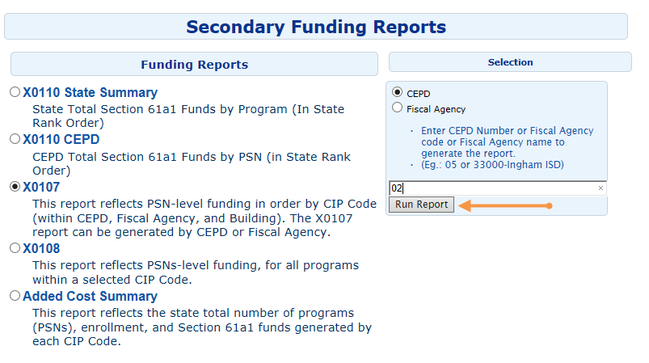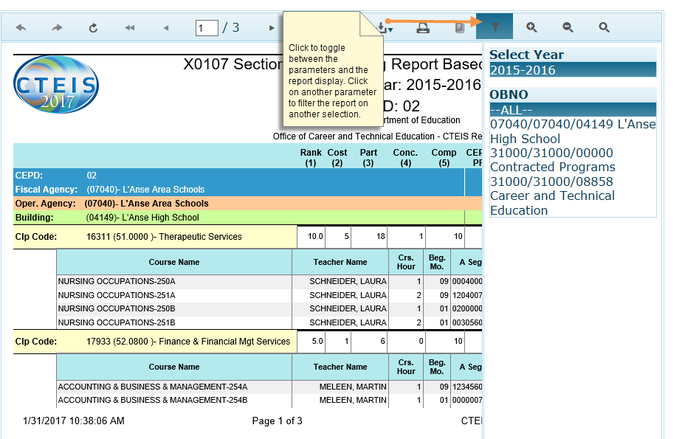Description: This report reflects PSN-level funding in order by CIP Code (within CEPD, Fiscal Agency, and Building). The X0107 report can be generated by CEPD or Fiscal Agency.

Location of Report: www.cteis.com, from the Menu, select Reports and then select Funding Reports. For Funding Reports for the years 2016 to present, select Funding Reports(2016+) and for the previous years' funding reports, select Funding Archives.

Report Levels:

• CEPD
• Fiscal AgencyExplanation of Program Descriptors, Rows, and Columns

Header Rows—CEPD, Fiscal Agency, and Building Totals

 Header Description CEPD a 2-digit code that identifies the Career Education Planning District in which the CTE program is located. This row reflects the CEPD total 60% and 40% Section 61a1 funds (as well as the CEPD Total 60% and 40% Program Formula Values – PFV). Fiscal Agency a 5-digit district code and name that identifies the fiscal agency of the CTE program. (Section 61a1 funds are distributed to the Fiscal Agency.) This row reflects the Fiscal Agency total 60% and 40% Section 61a1 funds (as well as the Fiscal Agency Total 60% and 40% Program Formula Values – PFV). Oper. Agency a 5-digit code and name that identifies the district that operates the CTE program. Building a 5-digit code and name that identifies the building in which the CTE program is located. This row reflects the Building total 60% and 40% Section 61a1 funds (as well as the Building Total 60% and 40% Program Formula Values – PFV).

CIP Code Rows (Program Totals) and Column Explanations

Columns Description
PSN Program Serial Number - a unique 5-digit code assigned to each individual state-approved CTE program. (The PSN appears to the left of the CIP Code.)
CIP Code Classification of Instructional Program Code – a 6-digit code assigned to each CTE program. (The CIP Code appears in parenthesis.)
Program Name identifies the program name of each CIP Code.
Rank (1) – Rank Factor (1, 2.5, 5, 10) this column reflects the rank factor (R) for each program, which is used in the formula to generate Section 61a1 funds. Only the top 20 programs on the State Rank List will receive a rank factor value greater than 1. Of the top 20 ranked programs, the top 7 programs have a rank factor of 10; the next 7 programs have a rank factor of 5; and the next 6 programs have a rank factor of 2.5. (All programs that fall below the top 20, have a rank factor of 1.)
Cost (2) – Cost Factor (1, 5, 10) this column reflects the cost factor (M) for each program, which is used in the formula to generate Section 61a1 funds. These cost factors are based on a 3-year average of the total expenditures per student, reported for each CIP Code – ranked from most expensive to least expensive. The top third of the programs (most expensive) have a cost factor of 10; the next third of the programs have a cost factor of 5; and the bottom third of the programs have a cost factor of 1.
Part. (3) Participants - the number of students who completed less than 7 segments of a program.  Participants (E) are weighted 1 in the Section 61a1 funding formula.
Conc. (4) Concentrators - the number of students who successfully completed 7 or more segments of a program (with a grade of 2.0 or better), but did not meet the requirements of a completer.  Concentrators (N) are weighted 5 in the Section 61a1 funding formula.
Comp (5) Completers – the number of students who successfully completed 12 segments of a program (with a grade of 2.0 or better), and took the technical skills assessment test, if applicable.  Completers (C) are weighted 10 in the Section 61a1 funding formula.
NoteThe student numbers reflected in the Participants, Concentrators, and Completers columns are used in the formula to generate Section 61a1 funds. The student numbers reflected in these three columns are unduplicated at the PSN level. (In other words, each student is only counted once per year for each program in which they were enrolled – as either a program participant, a program concentrator, or a program completer.) The progress of each student is determined at the end of the school year, based on the total number of segments successfully completed in each program.
60% PFV (6)60% Program Formula Value this column reflects the result of the following formula - for each program (PSN) that is in the top 20 CIP Codes on the State Rank List.
Formula: [(Ex1) + (Nx5) + (Cx10)] x M x R = Program Formula Value (PFV)
Explanation: [(Participants x 1) + (Concentrators x 5) + (Completers x 10)] x Cost Factor x Rank Factor = PFV
In order to calculate the amount of 60% Section 61a1 funds each program (PSN) will generate, the Program Formula Value (for each PSN) in this column, is divided by the State Total 60% Program Formula Value to produce a fraction. This fraction is then multiplied by the total amount of 60% funds (currently \$21,486,780) to calculate the dollar amount for each program PSN.
60% Funds (7) this column reflects the amount of 60% Section 61a1 funds generated by each program (PSN) that is in the top 20 CIP Codes on the State Rank List.
40% PFV (8)40% Program Formula Value this column reflects the result of the following formula - for each program (PSN) that was selected by the CEPD to generate 40% funds.
Formula: [(Ex1) + (Nx5) + (Cx10)] x M = Program Formula Value (PFV)
Explanation: [(Participants x 1) + (Concentrators x 5) + (Completers x 10)] x Cost Factor = PFV
Note: The 40% formula values are calculated by individual CEPD. In order to calculate the amount of 40% Section 61a1 funds each program (in a specific CEPD) will generate, each Program Formula Value in this column (for a specific CEPD) is divided by the CEPD Total 40% Program Formula Value (for that specific CEPD), to produce a fraction. This fraction is then multiplied by the CEPD Share Amount (for that specific CEPD) to calculate the dollar amount generated for each program (PSN) - in that specific CEPD.
40% Funds (9) this column reflects the amount of 40% Section 61a1 funds generated by each program (PSN) selected by the CEPD.
Total (10) this column reflects the total amount of Section 61a1 funds generated by each program (PSN).

Note:  It is possible that a program (PSN) will generate both 60% and 40% funds – as CEPDs may choose to support specific PSNs (that generate 60% funds) with their CEPD share (40%) funds.

Course-Section Rows and Column Explanations

Rows/Column Description
Course Name determined by the district.
TeacherName this column reflects the primary teacher (reported by the district) for each course-section.
Crs. Hour Course Hour determined by the district.
Beg. Mo Beginning Month – month the course-section (class) begins
A, B, C, D Segments reflects the specific segments of instruction being taught in each course-section (or sub-section). If all students in a course-section (class) are receiving the same segments of instruction, those segments will be reflected in the A Segments column. (Note: X = segment 10; Y = segment 11; and Z = segment 12)
Crs. Section Enroll (Dup.) reflects the total number of students enrolled in each course-section. Note: this column reflects duplicated numbers, in that students are counted for each course-section (class) during each term (semester) in which they are enrolled – within a program. (For example, if a student is enrolled for both the 1st and 2nd semester of a program, the student would be counted once in the 1st semester class, once in the 2nd semester class.) The numbers in this column are not used in the formula to generate Section 61a1 funds.